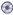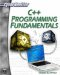# Chapter 4: FunctionsDownload CD Content

Chapter 1 provided a brief introduction to the concept of functions. A main function and the basic structure of a function were shown. This chapter will go into functions with much greater detail. You will learn how to create and use a variety of functions. In this chapter you will also be introduced to advanced concepts such as function overloading.

## Basic Structure

The first item to address is to explain exactly what a function is. A function is essentially a block of code that can be called by name, may be passed parameters, and may return a value. Another way to put that would be to say that a function is a group of related statements/expressions that work together to accomplish some goal and are grouped under a common name. Let’s take a look at the main function that all C and C++ programs must have. By dissecting it, you may get a better idea of what a function is and how one is built.

`int main() {   return 0; }`

The first line is called the function declaration line. It contains three major parts. Those parts are the return type, name, and parameter list. The return type simply tells you what kind of data this function will return. One way to think of it is to realize that you create functions to perform some goal. Whatever answer is generated from that function is what is returned. If you create a function to compute the area of a circle, then the return value would be the actual area computed. The return type for most functions can be any valid data type. It can be an int, float, bool, long, and so on. However, the main function must return an int. If the function does not return anything at all, then its return type is “void.”

 Hint! ANSI/ISO standards dictate that main must return an int. You will see some programmers return a void, but this is nonstandard. In this book, all main functions will return an int value. A return of 0 indicates that all is OK; a return of one indicates that something went wrong.

The next portion of the function declaration is the name. The primary function, the function that starts the program must be named main. Other functions can have any name you wish, provided the name follows the same conventions that variable names do. It is, however, a good idea to give the functions a name that indicates what they do. For example, if your function squares a number, give it a name such as the names you see here.

• square_num

• number_squared

• num_2

The last part of the declaration line is the parameter list. These are things you give the function. If, for example, your function squares a number, then you have to give it the number you want squared—that number is a parameter. You can have no parameters, one parameter, or several parameters. Also recall that another name for a parameter is an argument.

All of that makes up the declaration line of the function. What is left now is relatively simple. You have brackets { } enclosing the function, the statements/expressions that you wish the function to execute, and, finally, you return a value. Remember that brackets are used to denote boundaries around a block of code. Right now that block of code is a function. In later chapters you will see other blocks of code (such as loops and switch statements).

 Watchout! Remember that when returning a value, the value returned must, obviously, be of the same type that you declared as the function’s return type. If you declared the return type as int, you cannot use return 1.22, or return true, as your return statements. You will get a type mismatch error.C++ Programming Fundamentals (Cyberrookies)
ISBN: 1584502371
EAN: 2147483647
Year: 2005
Pages: 197
Authors: Chuck Easttom